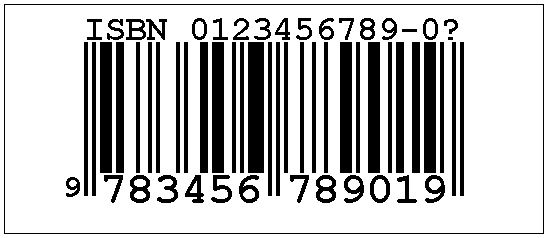# ISBN Encoding

The International Standard Book Number (ISBN) is based on an ISO International Standard that was first published in 1972 as ISO 2108. The number is also known as IBSN-10 as it consists of 10 digits. The new standard ISBN-13 is in the works and is planned to be used starting 2007.

An ISBN-10 consists of four parts which are separated by hyphen:

1. 1-4 digit country (language) indicator
2. Variable length Publisher code
3. Book number
4. 1 check digit.

Now there is where mathematics comes in. A valid ISBN d1d2...d10 is required to satisfy the following identity

 (1) 1×d1 + 2×d2 + 3×d3 + ... + 10×d10 = 0 (mod 11).

or, which is the same,

 (1') 10×d1 + 9×d2 + 8×d3 + ... + 1×d10 = 0 (mod 11).

The latter leads to a more direct computation of the last digit. If, as a result of (1') d10 comes out to be 10, in the ISBN it is replaced by an "X".

The applet below allows one to experiment with IBSN-10. The first nine digits of the ISBN string are clickable and modifiable. The last is calculated according to the algorithm (1'). The digits cycle through the values 0, 1, 2, 3, 4, 5, 6, 7, 8, 9, -. For a valid number, it is required that

1. the number of hyphens be three,
2. no two hyphens be in consecutive positions,
3. the penultimate symbol be hyphen.

For an invalid number, the last digit is replaced with "?".

### If you are reading this, your browser is not set to run Java applets. Try IE11 or Safari and declare the site https://www.cut-the-knot.org as trusted in the Java setup.What if applet does not run?

Presently, an ISBN-10 is encoded as an EAN-13 by prepending the ISBN Bookland code 978 and removing the hyphens, as shown at the bottom of the applet (these digits are not clickable). As a point of departure, the planned ISBN-13 will mimic ISBN-10's embedding into EAN-13 by prepending am ISBN-10 with "978-". Note that the check digits in ISBN and EAN are computed differently and independently. The "embedding" only relates to the first nine digits of an ISBN.

### References

1. E. B. Burger, M. Starbird, The Heart of Mathematics, Key College Publishing, 2000, p. 94